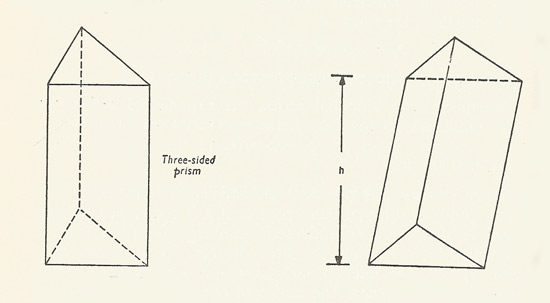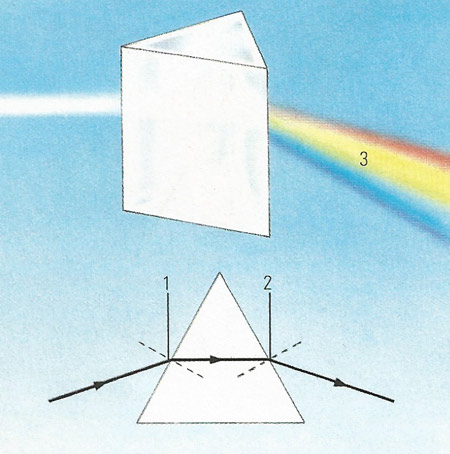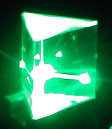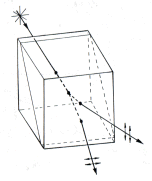A# prismFigure 1. A right three-side prism (left) and oblique prism (right).Figure 2. When light hits a prism it is refracted by the two surface it hits (1, 2). White light splits into the spectrum (3) because each of the colors of the spectrum have varying wavelengths. For example, the short wavelengths of blue and indigo are refracted more than the colors further down the spectrum with longer wavelengths such as orange and red.Figure 3. Schematic section through a Nicol prism. The original calcite rhombohedron is split along a diagonal plane, cemented back together again with Canada balsam, and then ground down to the desired shape. Optical polarimeters employ pairs of Nicol prisms.

In mathematics, a prism is a polyhedron constructed from two congruent n-sided polygons and n parallelograms. The word 'prism' comes from the Greek prizma, which relates to cutting or sawing. A prism is a semiregular polyhedron if all of its faces are regular polygons. If the lateral surfaces of the prism are perpendicular to the base, it is said to be a right prism; otherwise, it is known as a oblique prism (see Figure 1).

If the base of a prism is a regular n-agon, it is said to be a regular n-sided prism. The distance between the base and the upper surface is called the altitude. The volume of a prism with base-area B and altitude h is V = B.h.

A four-sided right prism is also called a right parallelepiped. A right parallelepiped whose altitude is equal to the edges of the base is a cube. A four-sided oblique prism is also called a parallelepiped. Prisms with equal altitudes have equal volumes.

A prismoid resembles a prism but has bases that are similar rather than congruent, and sides that are trapezoids rather than parallelograms. An example of a prismoid is the frustum of a pyramid. A prismatoid is a polyhedron with all its vertices lying in two parallel planes.

## Optical prisms

In physics, prismatic pieces of transparent materials are much used in optical instruments. In spectroscopes and devices for producing monochromatic light, prisms are used to produce dispersion effects, just as Newton first used a triangular prism to reveal that sunlight could be split up to give a spectrum of colors (see Figure 2). In binoculars and single-lens reflex cameras reflecting prisms (employing total internal reflection) are used in preference to ordinary mirrors. The Nicol prism is used to produce polarized light.### Nicol prism

A Nicol prism is an optical device for producing a beam of plane polarized light (see Figure 3). Two pieces of calcite crystal are cemented together with Canada balsam. Incident light is split into ordinary and extraordinary linearly polarized rays in the prism. The ordinary ray hits the balsam layer obliquely, and is totally internally reflected; the other ray emerges plane polarized for a certain range of incident angles.

The Nicol prism was invented by the British physicist William Nicol (1768–1851). It is used in the polarizing microscope, and also in geology to examine thin sections of rock microscopically.

### Wollaston prismThe Wollaston prism is a device, invented by William Wollaston, that separates randomly polarized or unpolarized light into two orthogonal linearly polarized beams that exit the prism at an angle determined by the wavelength of the light and the length of the prism. Wollaston prisms are used in some kinds of polarimeter.